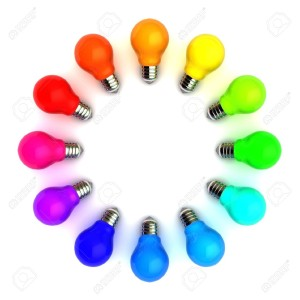# Bulbs in circle puzzle

1,290.8K Views

On a circle there are 2014 light bulbs, 2 are ON, and 2012 are OFF.

You can choose any bulb and change the neighbor’s state i.e. ON to OFF or OFF to ON.

Doing so,
What is the maximum number of bulbs we can turn on?ANSWER–  All the 2014 can be turned on

EXPLANATION-

When we Keep two lighted bulbs adjacent.

Let’s assume initial state of circuit as {0,0,0,1,1,0,0,0,0…..,0,0}                                                    where 1 = Switched ON & 0 = Switched OFF

Choose second last bulb from bulb which has state =1, then 2 bulb will be lighted on either sides
next state becomes {1,0,1,1,1,1,0,1,0,0,0…..0,0}
So, at each step we are lighting 4 bulbs, since 2012 is multiple of 4, there will come a stage where all bulbs will be ON.

I think it depends on the number of lightbulbs and the order the two lighted bulbs are in.

4 cases
1. If the two lighted bulbs are next to each other and the total number of lightbulbs is an odd multiple of 2 (odd # * 2), then we can turn on all bulbs.
2. If the two lighted bulbs are next to each other and the total number of lightbulbs is an even multiple of 2 (even # * 2), then we can turn on all but 2, **unless the total number of bulbs is 2, which doesn’t make sense here as a scenario anyways.
3. If the two lighted bulbs have a one bulb gap between them, and the total number of lightbulbs is an odd multiple of 2 (odd # *2), then we can turn on all but 2.
4. If the two lighted bulbs have a one bulb gap between them, and the total number of lightbulbs is an even multiple of 2 (even # * 2), then we can turn on all bulbs.

The two cases relevant here are case #2 and case #4. Try this for the simplest cases: 4 light bulbs and 6 light bulbs, and you’ll quickly see that these conclusions should hold true for larger sets.

One. Note that it says “change the neighbor’s state” NOT “neighbors’ stateS”. It does not say that changing state from 0-1 has an impact on the next neighbor to iterate.

The question in not clear enough.  Because when sayin that: You can choose any bulb and change the neighbor’s state i.e. ON to OFF or OFF to ON, does it mean that I must change both neighbors or can I choose to change only one of them? Also it is not clear if when asking “What is the maximum number of bulbs we can turn on?” means also how many will stay turned on or only how many can be turned on?

Lets check all the cases:
If we must change the state of both the neighbors than we have 3 cases
1) the bulbs are next to each other like 001100 than we can light all the bulbs but only 2 will stay on
2) If there is a gap of one between the 2 bulbs like 010100 than we can light all the bulbs but only 4 will stay on
3) If the gap is greater than 2 all the bulbs can be light but only 6 will stay on
If we don’t have to change the state of all the neighbors than we can light all the bulbs  and all of them will stay on.